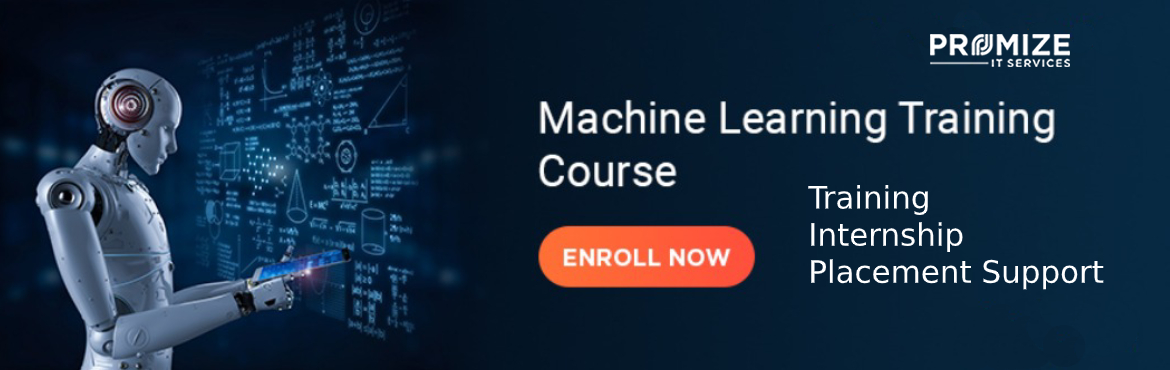# Attend Machine Learning DEMO On Friday 27th Dec @ 10 AM

• ## Training Fee

Sale Date Ended

INR 25000
Sold Out

#### Invite friends

Page Views : 19

➢      Introduction

➢      Python Revisited and Tools for Development

-         Anaconda , Jupyter Notebook

➢     Machine Learning – Introduction

-          Supervised and Unsupervised Learning.

-          bias -variance dichotomy

➢     Python Lib for Mathematical Ops (NumPy)

-          NumPy Overview

-          Properties, Purpose, and Types of ndarray

-          Class and Attributes of ndarray Object

-          Basic Operations: Concept and Examples

-          Accessing Array Elements: Indexing, Slicing, Iteration, Indexing with Boolean Arrays

-          Copy and Views

-          Shape Manipulation

-          Linear Algebra

-          Code Exercises

➢     Scientific Computing with Python

-          SciPy

-          SciPy sub-packages

-          Subpackages: cluster, fftpack , linalg , signal, integrate, optimize, stats

-          Code Exercises

➢     Data Manipulation with Python (Pandas)

-          Introduction to Pandas Data Structures

-          Series

-          Dataframe

-          Missing Values and Data Cleansing

-          Data Operations

➢     Statistical and Mathematical operations

-          Matrix operations, Probability Theory(Bayes' Theorem)

-          Statistical knowledge for ML- Mean, Median, Mode , Z-scores,

-          Hypothesis Testing

-          Chi-Square Test

➢     Data Visualization (Matplotlib)

-          Plotting Graphs and Charts

-          Matplotlib Features:

✓ Line Properties Plot with (x, y)

✓ Controlling Line Patterns and Colors

✓ Set Axis, Labels, and Legend Properties

✓ Alpha and Annotation

✓ Multiple Plots

✓ Subplots

➢     Machine Learning with Python (Scikit–Learn)

-          ML Glossary- Variable types, k-fold CV, AUC ,F1 score, Overfitting /Underfitting, Generalization, Data split & hyper parameter training

-          Supervised learning – Regression

-          Different types of Regression - Linear and Logistic

-          Decision tree Algorithms

-          Supervised Learning- Classification

-          KNN Classification

-          Clustering- Introduction , k-means clustering

-          Code Exercises